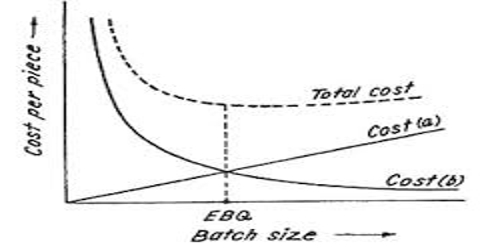# Economic Batch Quantity (EBQ)

Economic batch quantity (EBQ) also called optimal batch quantity or economic production quantity is a measure used to determine the number of units that can be produced at minimum average costs in a given batch or production run. The Economic Batch Quantity model, or Production lot-size model, is similar to the EOQ model in that art optimum is to be calculated for the batch quantity to be produced. It is the order size of a production batch that minimizes the total cost. Hence, the batch cost is used to determine the cost per unit or article per unit.

According to Basu & Das – “EBQ is the Optimum batch size for the manufacture of an item or component, at the lowest cost. It is also mentioned that the batch size is a tradeoff between unit costs that increase with butch size and those that decrease.”

In the words of Charles T.Horngren –

“EBQ is an inventory-related equation that determines the optimum order quantity that a company should hold in its inventory given a set cost of production, demand rate, and other variables.”

Finally, economic batch quantity example is a technique to control the cost of a batch like EN for controlling material cost.

Calculation of EBQ

The Economic Batch Quantity (EBQ) is worked out by applying the following formula:

EBQ = √[(2*U*S) / CC]

Where U = Total number of units to be produced in a year,

S = Set-up cost per batch

CC = Carrying cost per unit of production for one year.

If the rate of interest and cost of the production per unit is given, the formula may be amended as under:

EBQ = √[(2*U*S) / (I * Cm]

Where I = Interest per Taka per year

Cm = Coat per manufacture per unit.